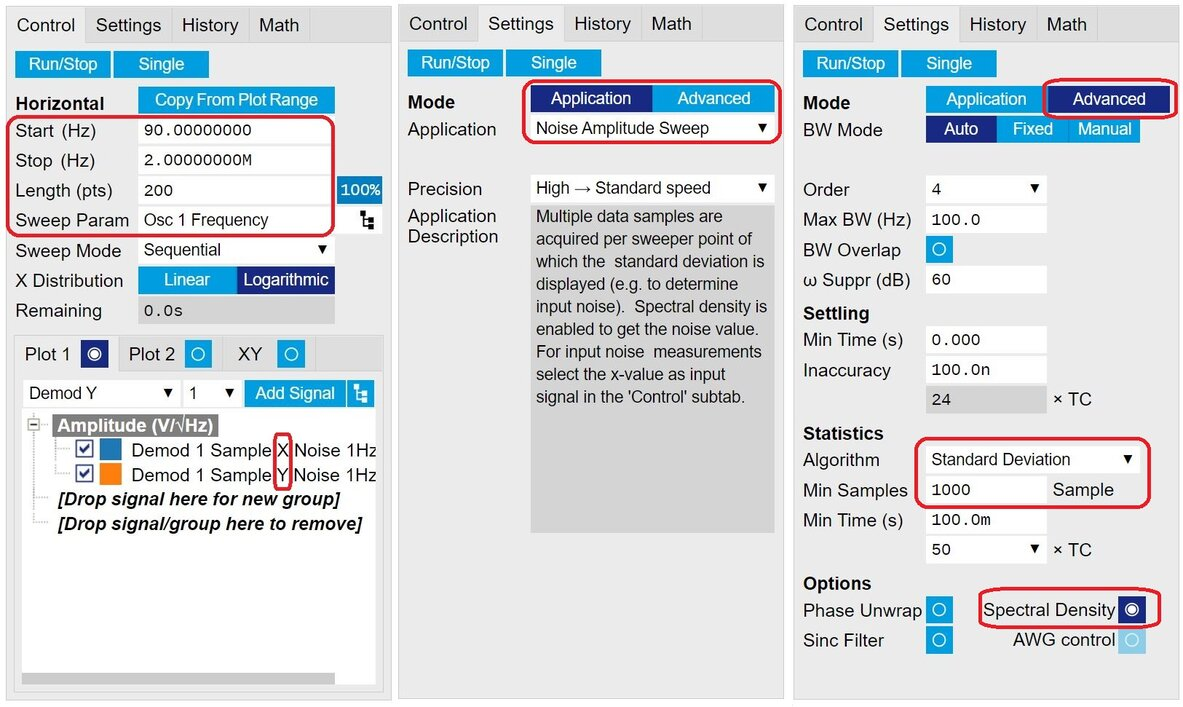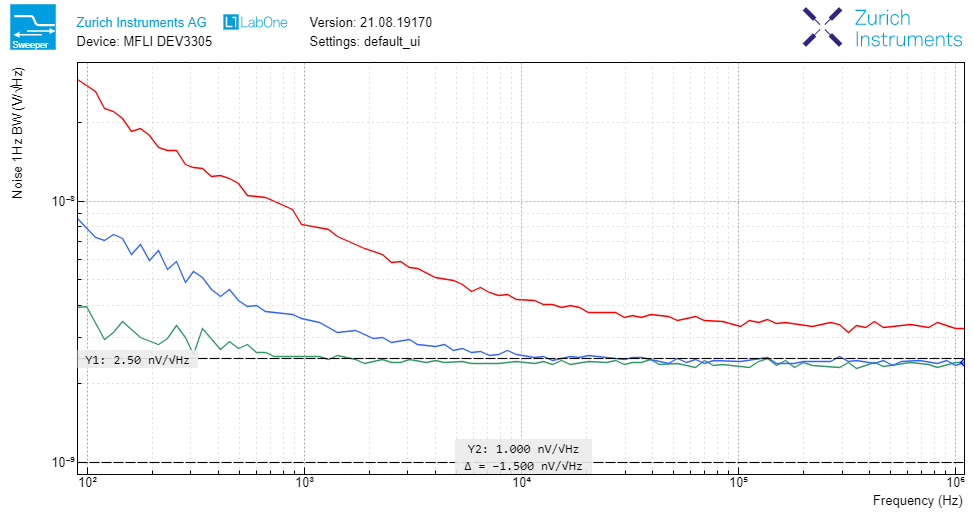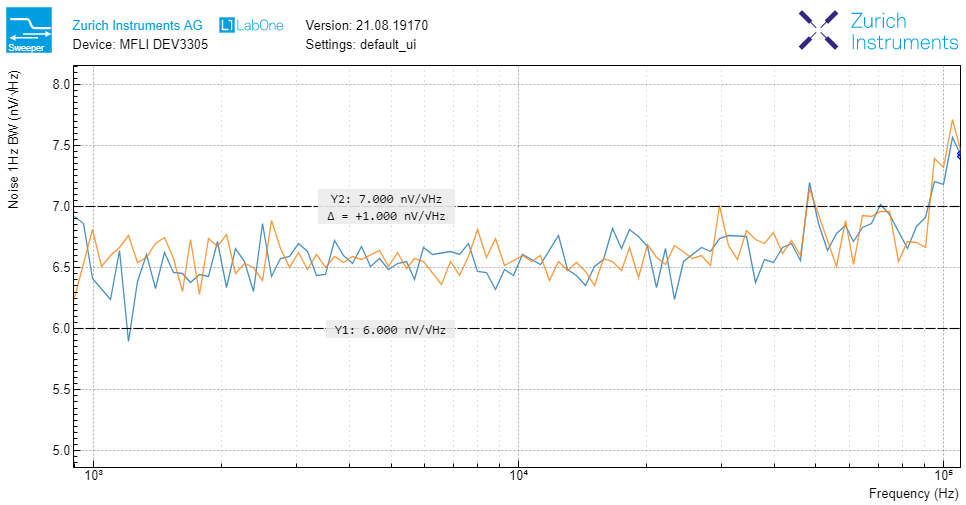# Noise Spectral Density Measured with Lock-in Amplifiers

August 15, 2021 by Mehdi Alem

## Introduction

Proper noise characterization is essential in many applications, especially when dealing with tiny signals buried in noise like in nanotechnology, photonics, and quantum physics. Noise characteristics of a system are often represented by the noise spectral density in amplitude mode, expressed in terms of $$\text{V}/\sqrt{\text{Hz}}$$, or in power mode, called power spectral density (PSD) and expressed in $$\text{V}^2/\text{Hz}$$, within the frequency range of interest. It is crucial to measure the noise of a system with high accuracy and precision, as it will otherwise lead to an incorrect system design or a wrong estimate of system behavior. Zurich Instruments' lock-in amplifiers offer simple and efficient approaches to noise characterization thanks to the extremely low noise of their hardware as well as the built-in software tools for noise measurement. The LabOne instrument control and data acquisition software offers three methods for noise spectrum measurement:

• Oscilloscope FFT
• Spectrum Analyzer
• Frequency Sweeper

The FFT tool embedded in the scope module of LabOne calculates the spectrum of the input signal before applying demodulation on the signal. In contrast, the spectrum analyzer tool of LabOne obtains the Fourier transform of acquired signals after demodulation. While the Scope FFT provides a wide frequency range, the Spectrum Analyzer offers a very high frequency resolution. Nevertheless, both approaches use the FFT algorithm to compute signal spectra. Therefore, they may suffer from the drawbacks of FFT calculations such as spectral leakage, limited number of signal points, etc.

In contrast to the scope and spectrum tools, the sweeper module of LabOne measures the spectral component of a signal directly without applying the FFT operation. This approach avoids all the calculation artifacts that might pollute the results due to FFT algorithm. On the other hand, it offers the advantages of scope and spectrum tool together, i.e. a wide frequency range with a high frequency resolution. Compared to the other two methods, the sweeper technique comes at the cost of longer measurement time. Therefore, in applications where accurate and precise noise characterization is required, the Sweeper module of LabOne is a perfect choice; however, approximate but fast noise measurements can be carried out by Scope FFT or Spectrum Analyzer depending on required frequency range and resolution.

The focus of this blog post is on the frequency sweeper approach to noise spectrum measurement. In the next section, we will analyze the mathematical background of noise measurement in lock-in amplifier and how to relate the noise at the input of an instrument to the demodulated components obtained by lock-in detection. The final section is dedicated to a hands-on experiment of noise measurement using the frequency sweeper of MFLI Lock-in Amplifier.

## Mathematical Background

In this section, we discuss the theory behind the sweeper approach for noise measurement. At the end of this analysis, we should have a clear understanding how the bandpass noise at the input of a lock-in amplifier is obtained from the baseband signal components measured by the lock-in amplifier.

### Noise representation

Suppose that $$n(t)$$ is the noise you are interested to measure. The source of this noise can be a device under test (DUT) connected to the input of a lock-in amplifier or the input noise of the instrument when its input port is grounded by a short-circuit cap. Using a lock-in amplifier we can measure the noise component at a specific frequency and then the sweeper varies the measurement frequency to obtain the entire noise spectrum. Assume that $$n_\omega(t)$$ is the noise component at frequency $$\omega$$. Therefore, it can be written as a sinusoidal signal whose amplitude and phase are random variables. $n_\omega(t)=\sqrt{2}R \sin(\omega t+\theta)$ where $$R$$ is the RMS (root-mean square) amplitude noise and $$\theta$$ is the phase noise at frequency $$\omega$$. The numerical factor $$\sqrt{2}$$ is used by convention to represent the amplitude in RMS instead of peak value. A dual-phase lock-in amplifier measures the in-phase component $$X$$ and the quadrature component $$Y$$ which can be obtained by expanding the above expression as follows. $n_\omega(t)=\sqrt{2} X \sin(\omega t) + \sqrt{2} Y \cos(\omega t)$ in which, $X= R\cos(\theta)\\ Y=R\sin(\theta)$ The noise components $$X$$ and $$Y$$ are independent random variables with zero-mean Gaussian (normal) distributions; while the amplitude $$R$$ and phase $$\theta$$ are Rayleigh- and uniform-distributed variables, respectively (as also explained here). Next, we will see how the measured components $$X$$ and $$Y$$ at frequency $$\omega$$ can be related to the noise power at this frequency.

### Noise power

The instantaneous power of noise at frequency $$\omega$$ is obtained by squaring the noise signal $$n_\omega(t)$$ as calculated below. $p_\omega(t) = n_\omega^2(t) = 2R^2 \sin^2(\omega t+\theta)$ Alternatively, the noise power at frequency $$\omega$$ can be expressed in terms of in-phase and quadrature representation of the noise by the following expression. $p_\omega(t) = 2X^2 \sin^2(\omega t) + 2Y^2 \cos^2(\omega t) + 2XY\sin(2\omega t)$ The time-averaged noise power at frequency $$\omega$$ is obtained by taking the time average of the instantaneous noise power over one or multiple periods $$T=2\pi/\omega$$ as computed below. $P_\omega = \frac{1}{T}\int_{T}{p_\omega(t)\ dt}$ Time averaging is basically performed by demodulation which includes homodyne mixing and low-pass filtering. Substituting $$p_\omega(t)$$ in the above equation, we will obtain the following average noise power at frequency $$\omega$$ in terms of signal amplitude $$R$$ and quadrature components $$X$$ and $$Y$$. $P_\omega = R^2 = X^2 + Y^2$ Since the signal components are random variables, we need to take the ensemble average of the time-averaged power to obtain the final noise power $$P_n$$ at a certain frequency. We represent ensemble averaging by the mathematical mean operation $$\mathbb{E}$$ to obtain the noise power as follows. $P_n =\mathbb{E}\{ P_\omega \} = \mathbb{E}\{R^2\} = \mathbb{E}\{X^2\} + \mathbb{E}\{ Y^2 \}$ The in-phase and quadrature components are zero-mean random variables and thus their second moment can be replaced by their variance, i.e. $$\sigma_X^2$$ and $$\sigma_Y^2$$, respectively. However, the amplitude component $$R$$ has a non-zero mean $$\mu_R$$ which contributes to the its second moment. This can be summarized as follows. $P_n =\mu_R^2 + \sigma_R^2 =\sigma_X^2 + \sigma_Y^2$ It should be noted that both mean and variance of amplitude noise contribute to the noise power. People often measure only the variance and forget about the mean value. This results in an inaccurately smaller noise spectrum. Hence, we should always use the quadrature components $$X$$ and $$Y$$, instead of the amplitude component $$R$$, for noise characterization. Further details in this regard are available in this blog post.

### Noise spectrum

The noise power spectral density (PSD) is obtained by dividing the noise power by the measurement bandwidth which is the noise equivalent power (NEP) bandwidth of the bandpass filter around the noise frequency $$\omega$$. However, the lock-in amplifier uses a low-pass filter (LPF) with NEP bandwidth $$B$$ for measurement. The equivalent bandwidth for the corresponding band-pass filter (double sideband) is twice the LPF bandwidth, i.e. $$W=2B$$, as demonstrated in . Therefore, the noise power spectral density $$S_n$$ expressed in $$\text{V}^2/\text{Hz}$$ is obtained as follows. $S_n = \frac{P_n}{W} = \frac{\sigma_X^2 + \sigma_Y^2}{2B} = \frac{1}{2}\bigg(\frac{\sigma_X^2}{B} + \frac{\sigma_Y^2}{B}\bigg) = \frac{1}{2}(S_X + S_Y)$ where $$S_X$$ and $$S_Y$$ are the PSD of noise components $$X$$ and $$Y$$, respectively. Our goal is to measure the noise spectral density at the input of the instrument, i.e. $$S_n$$; however, the lock-in amplifier can measure the spectral density of in-phase and quadrature components of the noise, i.e. $$S_X$$ and $$S_Y$$. The above equation simply says that to obtain the final noise spectrum we need to take the mean of the noise spectra of signal components.

In almost all applications, in-phase and quadrature components have an equal noise power and thus, we can simply measure either of the signal components, i.e. $$S_n=S_X=S_Y$$. It should be noted that in some rare applications such as measuring the squeezed states of light where the quadrature components have different noise levels, it is important to take the mean of noise spectra.

Another important point to notice is the fact that averaging should be taken over the power spectral density expressed in $$\text{V}^2/\text{Hz}$$ and not over the amplitude spectral density represented by $$\text{V}/\sqrt{\text{Hz}}$$. Otherwise, the final spectrum will be slightly less than the actual one. The amplitude spectral density is simply obtained by taking the square root of power spectral density.

## Frequency Sweeper

The Sweeper module of LabOne can sweep a device parameter, in this case an oscillator frequency, and then perform signal acquisition for each parameter value to obtain the spectrum of measured signal. Depending on the signal frequency, the sweeper automatically adjusts the measurement bandwidth to keep a balance between the measurement accuracy and speed. As explained in the previous section, the key point in noise measurement by lock-in amplifiers is to acquire the in-phase and quadrature components $$X$$ and/or $$Y$$ instead of $$R$$.

As a first step for noise characterization, we need to obtain the input noise of the lock-in amplifier. As explained in this blog post, you need to attach a short-circuit cap to the input port of the instrument to ground the signal path. Then, you should adjust the settings of the Sweeper module to perform an optimal noise measurement as depicted in Figure 1.Figure 1: Red boxes highlight the settings of the LabOne Sweeper module required for a proper noise measurement. (Click to zoom)

First of all, the sweeper should subscribe to the in-phase and quadrature components of the demodulated signal. Then, the 'Application' mode should be set to 'Noise Amplitude Sweep'. This mode optimizes the settings in the 'Advanced' mode to perform a precise noise measurement. Specifically, it enables the 'Spectral Density' button to divide the measured signal by the noise equivalent power (NEP) bandwidth of the demodulation LPF. Moreover, it utilizes the 'Standard Deviation' algorithm for statistical calculation to obtain the noise power. Figure 2 shows the input noise of an MFLI for 3 different input ranges, i.e. 1 mV, 30 mV, and 100 mV. Since in-phase and quadrature components have identical noise levels, here it suffices to only measure $$\sqrt{S_X}$$ to obtain the noise spectral density $$\sqrt{S_n}$$ as explained above.Figure 2: Input noise of the MFLI obtained with the Sweeper module of LabOne within four frequency decades from 100 Hz to 1 MHz for three input ranges: 1 mV (green), 30 mV (blue), and 100 mV (red). It demonstrates an input noise as low as 2.5 nV/rtHz.

Figure 2 clearly demonstrates that by expanding the input range of the instrument, the input noise increases. This indicates that we should always use the minimum input range that covers the entire swing of an input signal. To do so, the lock-in amplifier is equipped with the 'Auto Range' feature to optimize the input range depending on the signal or noise level. The lowest input noise floor of the MFLI is around $$2.5\ \text{nV}/\sqrt{\text{Hz}}$$; however, to achieve lower noise levels we can benefit from the cross-correlation technique as demonstrated in this application note. Additionally, in Figure 2 it is possible to distinguish two types of noise: pink or 1/f noise at low frequencies below a corner point and white or flat noise for high frequencies above the corner point.

### Thermal noise

To verify the accuracy and precision of the noise spectral density measurement using lock-in amplifiers, we can obtain the noise of a resistor and compare it with its theoretical thermal noise expressed by the following expression : $S_n = 4k_\text{B} T R$ where $$T$$ is the absolute temperature of the resistor and $$R$$ is its resistance. $$k_\text{B}$$ denotes the Boltzmann constant. For a resistor of 2.2 kΩ at room temperature, the noise spectral density $$\sqrt{S_n}$$ is about $$6$$ to $$7\ \text{nV}/\sqrt{\text{Hz}}$$. Figure 3 shows the noise spectral density of such a resistor measured by the MFLI within a frequency range from 1 kHz to 100 kHz.Figure 3: Thermal noise spectral density of a 2.2 kΩ resistor measured by the MFLI and acquired by the Sweeper module of LabOne. It clearly shows that in-phase and quadrature components have a similar noise level.

The flatness of the measured spectrum within two frequency decades is a clear indication of white thermal noise accurately and precisely measurement by the frequency Sweeper of Zurich Instruments' lock-in amplifiers. It should be noted that the two wire leads of the resistor is directly connected to the core and shield of the BNC port of the MFLI signal input and no driving signal is required. Moreover, the input impedance of the instrument should be set at its high value, in this case 10 MΩ. Otherwise, the measurement outcome is skewed by the low input impedance of the device.

## Conclusions

This post focuses on how to measure noise spectral density using a lock-in amplifier with emphasis on the frequency sweep approach because of its advantages such as high accuracy and precision as well as its wide frequency range and resolution. A mathematical analysis relates the noise at the input of a lock-in amplifier to the demodulated signal components. Finally in a practical experiment, we measure the thermal noise of a resistor by the MFLI demonstrating a good agreement with the expected value from the theory.

## References

1. John G. Proakis and Masoud Salehi, Digital Communications. 5th ed., McGraw-Hill, 2008.
2. Wikipedia: Johnson–Nyquist noise